## Reduction of Order

Some second‐order equations can be reduced to first‐order equations, rendering them susceptible to the simple methods of solving equations of the first order. The following are three particular types of such second-order equations:

Type 1: Second‐order equations with the dependent variable missing

Type 2: Second‐order nonlinear equations with the independent variable missing

Type 3: Second‐order homogeneous linear equations where one (nonzero) solution is known

Type 1: Second‐order equations with the dependent variable missing. Examples of such equations include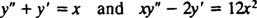The defining characteristic is this: The dependent variable, y, does not explicitly appear in the equation. This type of second‐order equation is easily reduced to a first‐order equation by the transformationThis substitution obviously implies y″ = w′, and the original equation becomes a first‐order equation for w. Solve for the function w; then integrate it to recover y.

Example 1: Solve the differential equation y′ + y″ = w.

Since the dependent variable y is missing, let y′ = w and y″ = w′. These substitutions transform the given second‐order equation into the first‐order equation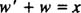which is in standard form. Applying the method for solving such equations, the integrating factor is first determined,and then used to multiply both sides of the equation, yieldingTherefore,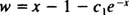Now, to give the solution y of the original second‐order equation, integrate:This gives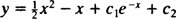Referring to Theorem B, note that this solution implies that y = c 1 e − x c 2 is the general solution of the corresponding homogeneous equation and that y = ½ x 2 – x is a particular solution of the nonhomogeneous equation. (This particular differential equation could also have been solved by applying the method for solving second‐order linear equations with constant coefficientes.)

Example 2: Solve the differential equation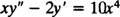Again, the dependent variable y is missing from this second‐order equation, so its order will be reduced by making the substitutions y′ = w and y″ = w′:which can be written in standard form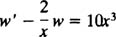The integrating factor here iswhich is used to multiply both sides of the equation, yieldingIntegrating w gives yLetting c 1 = ⅓ c 1, the general solution can be writtenExample 3: Sketch the solution of the IVP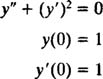Although this equation is nonlinear [because of the term ( y′) 2; neither y nor any of its derivatives are allowed to be raised to any power (other than 1) in a linear equation], the substitutions y′ = w and y″ = w′ will still reduce this to a first‐order equation, since the variable y does not explicitly appear. The differential equation is transformed intowhich is separable: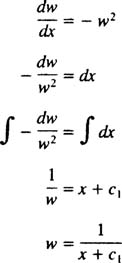Since y′ = w, integrating gives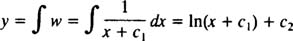Now apply the initial conditions to determine the constants c 1 and c 2.Because c 1 = 1, the first condition then implies c 2 = 1 also. Thus the solution of this IVP (at least for x > −1) is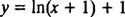whose graph is shown in Figure .Figure 1

Type 2: Second‐order nonlinear equations with the independent variable missing. Here's an example of such an equation:The defining characteristic is this: The independent variable, x, does not explicitly appear in the equation.

The method for reducing the order of these second‐order equations begins with the same substitution as for Type 1 equations, namely, replacing y′ by w. But instead of simply writing y″ as w′, the trick here is to express y″ in terms of a first derivative with respect to y. This is accomplished using the chain rule:Therefore,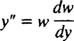This substitution, along with y′ = w, will reduce a Type 2 equation to a first‐order equation for w. Once w is determined, integrate to find y.

Example 4: Solve the differential equation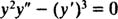The substitutions y′ = w and y″ = wdw/dy) tranform this second‐order equation for y into the following first‐order equation for w:Therefore,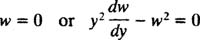The statement w = 0 means y′ = 0, and thus y = c is a solution for any constant c. The second statement is a separable equation, and its solution proceeds as follows:Now, since w = dy/dx, this last result becomeswhich gives the general solution, expressed implicitly as follows: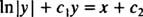Therefore, the complete solution of the given differential equation isType 3: Second‐order homogeneous linear equations where one (nonzer) solution is known. Sometimes it is possible to determine a solution of a second‐order differential equation by inspection, which usually amounts to successful trial and error with a few particularly simple functions. For exmple, you might discoer that the simple function y = x is a solution of the equation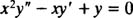or that y = x satisfies the equationOf course, trial and error is not the best way to solve an equation, but if you are lucky (or practiced) enough to actually discover a solution by inspection, you should be rewarded.

If one (nonzero) solution of a homogeneous second‐order equation is known, there is a straightforward process for determining a second, linearly independent solution, which can then be combined wit the first one to give the general solution. Let y 1 denote the function you know is a solution. Then let y = y 1 vx), where v is a function (as yet unknown). Substitute y = y 1 v into the differential equation and derive a second‐order equation forv. This will turn out to be Type 1 equation for v (because the dependent variable, v, will not explicitly appear). Use the technique described earlier to solve for the function v; then substitute into the expression y = y 1 v to give the desired second solution.

Example 5: Give the general solution of the differential equation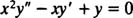As mentioned above, it is easy to discover the simple solution y = x. Denoting this known solution by y 1, substitute y = y 1 v = xv into the given differential equation and solve for v. If y = xv, then the derivatives are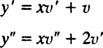Substitution into the differential equation yields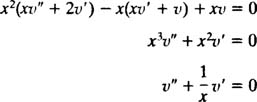Note that this resulting equation is a Type 1 equation for v (because the dependent variable, v, does not explicitly appear). So, letting v′ = w and v” = w′, this second‐order equation for v becomes the following first‐order eqution for w: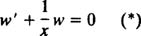The integrating factor for this standard first‐order linear equation is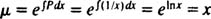and multiplying both sides of (*) by μ = x givesIgnore the constant c and integrate to recover v: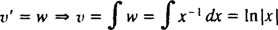Multiply this by y 1 to obtain the desired second solution,The general solution of the original equation is any linear combination of y 1 = x and y 2 = x In | x|:This agrees with the general solution that would be found if this problem were attacked using the method for solving an equidimensional equation.

Example 6: Determine the general solution of the following differential equation, given that it is satisfied by the function y = e x :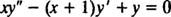Denoting the known solution by y 1 substitute y = y 1 v′ = e x v into the differential equation. With y = x, the derivatives areSubstitution into the given differential equation yields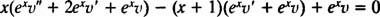which simplifies to the following Type 1 second‐order equation for v: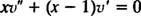Letting v′ = w, then rewriting the equation in standard form, yields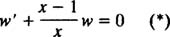The integrating factor in this case is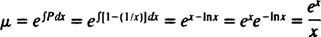Multiplying both sides of (*) by μ μ = x x yields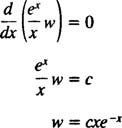Ignore the constant c and integrate to recover v: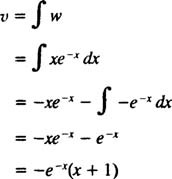Multiply this by y 1 to obtain the desired second solution,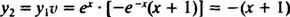The general solution of the original equation is any linear combination of y 1 and y 2Back to Top
A18ACD436D5A3997E3DA2573E3FD792A